## LetsPlayMaths.Com

WELCOME TO THE WORLD OF MATHEMATICS

# Class 6 Integers

Introduction to Integers

Integers on the Number Line

Ordering of Integers

Absolute Value of an Integer

Addition of Integers Using Number Lines

Subtraction of Integers Using Number Lines

Subtraction of Integers

Integers Test

Integers Worksheet

## Introduction to Integers

Set of positive numbers, zero and negative numbers is known as integers.

..., −5, −4, −3, −2, −1, 0, 1, 2, 3, 4, 5, ...

1, 2 , 3, 4, 5, ... are knwon as positive integers.

−1, −2, −3, −4, −5 are known as negative integers

The number zero is an integer. It is neither positive nor negative.

## Integers on the Number Line

Let's draw a line and mark a point in the middle of it. We number this point with zero.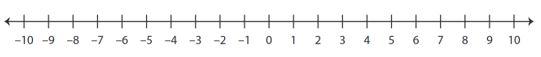We mark equal distances on the right as well on the left of zero. On the right side of zero label the points with numbers 1, 2, 3, 4, 5, ... and on the left side of the zero label the points with numbers −1, −2, −3, −4, −5, ....

So, we can represent all the integers on a number line as shown in the above figure.

## Ordering of Integers

Between two given integers, the integer occurring on the right side on the number line is greater than that occurring on the left side.

For Example.

5 > 2, since 5 is to the right of 2.

3 > 0, since 3 is to the right of 0.

0 > −2, since 0 is to the right of −2.

−5 > −8, since −5 is to the right of −8.

Some basic rules are provided below.

1. Every positive integer is greater than zero.

2. Zero is greater than every negative integer.

3. Every positive integer is greater than every negative integer.

## Absolute Value of an Integer

The absolute value of an integer 'p' is the numerical value of 'p' regardless of it's sign. We denote the absolute value of 'p', by |p|. Let's see some examples.

Example 1. Find the absolute value of −5.

Solution. |−5| = 5.

Example 2. Find the absolute value of 12.

Solution. |12| = 12.

Example 3. Find the value of |−12 + (−15)|.

Solution. |−12 + (&minus15)| = |−12 −15)| = |−27| = 27.

Example 4. Find the value of |25 − (−45 + 15)|.

Solution. |25 − (−45 + 15)| = |25 − (−30)| = |25 + 30| = |55| = 55

## Addition of Integers Using Number Lines

We have already known how to add two whole numbers using number lines. Let's see some examples.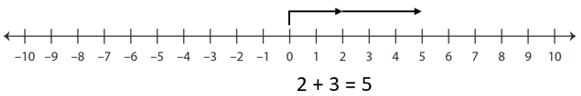Let's extend this method of addition of whole numbers to the integers.

Example 1. Add −2 and 4.

Solution. On the number line, we start from −2 and move 4 steps to the right of −2.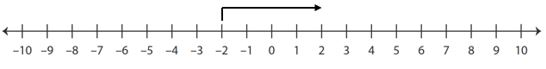We end up at +2 or 2.
Therefore, −2 + 4 = 2.

Example 2. Add 6 and −9.

Solution. On the number line, we start from 6 and move 9 steps to the left of 6.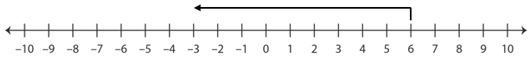We end up at −3.
Therefor, 6 + (−9) = −3.

Example 3. Add −3 and −6.

Solution. On the number line, we start from −3 and move 6 steps to the left of −3.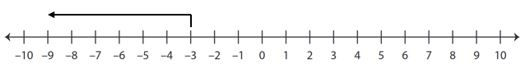We end up at −9.
Therefore, −3 + (−6) = −9.

## Addition of two like integers

When integers have same signs, we add their absolute values and assign the same sign to the sum. Let's see some examples.

Example 1. Add + 4 and +5.

Solution. (+4) + (+5) = + {|+4| + |+5|} = + {4 + 5} = +9

Example 2. Add −5 and −8.

Solution. (−5) + (−8) = − {|−5| + |−8|} = − {5 + 8} = −13

When integers have different signs, we determine the difference of their absolute values, and assign the sign of the integer having greater absolute value. Let's see some examples.

Example 1. Add −7 and 19.

Solution. Absolute value of −7 = |−7| = 7

Absolute value of 19 = |+19| = 19

Difference of absolute values is 19 − 7 = 12

Since the integer with greater absolute value is +19, and it's sign is +.

(−7) + (+19) = +12

Example 2. Add −25 and +5.

Solution. Absolute value of −25 = |−25| = 25

Absolute value of +5 = |+5| = 5

Difference of absolute values is 25 − 5 = 20

Since the integer with greater absolute value is −25, and it's sign is −.

(−25) + (+5) = −20

1. If 'a' and 'b' are any two integers, then a + b is also an integer.

2. If 'a' and 'b' are any two integers, then a + b = b + a.

3. If 'a', 'b' and 'c' are any three integers, then a + (b + c) = (a + b) + c

4. If 'a' is in integer, then a + 0 = 0 + a = a.

5. The sum of any integer and it's opposite integer (known as additive inverse) is zero. a + (−a) = 0

6. If a > b, then a + c > b + c and if a < b, a + c < b + c, where a, b, c are any integers.

## Subtraction of Integers Using Number Lines

To subtract a positive integer, we must move to the left on the number line. Let's see some examples.

Example 1. Subtract 5 from 3.

Solution. On the number line, we start from 3 and move 5 steps to the left of 3.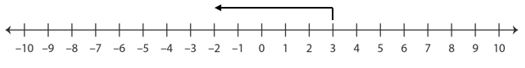We end up at −2.
Therefore, 3 − 5 = −2.

Example 2. Subtract 6 from −2.

Solution. On the number line, we start from −2 and move 6 steps to the left of −2.We end up at −8.
Therefore, −2 − 6 = −8.

To subtract a negative integer, we must move to the right on the number line. Let's have a look at some examples.

Example 1. Subtract −4 from 6.

Solution. On the number line, we start from 6 and move 4 steps to the right of 6.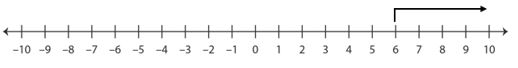We end up at 10.
Therefore, 6 − (−4) = 6 + 4= 10.

Example 2. Subtract -5 from -4.

Solution. On the number line, we start from -4 and move 5 steps to the right of -4.We end up at 1.
Therefore, −4 − (−5) = −4 + 5= 1.

## Subtraction of Integers

Subtraction of one integer from another integer is same as adding the additive inverse of the integer that is being subtracted to the other integer. Let's see some examples.

Example 1. Subtract 6 from 15.

Solution. Additive inverse of 6 is −6.
15 − 6 = 15 + (−6) = 9.

Example 2. Subtract 5 from −7.

Solution. Additive inverse of 5 is −5.
−7 − 5 = −7 + (−5) = −12.

Example 3. Subtract −232 from −165.

Solution. −165 − (−232) = −165 + 232 = 67

## Class-6 Integers Test

Integers Test - 1

Integers Test - 2

## Class-6 Integers Worksheet

Integers Worksheet - 1

Integers Worksheet - 2

Integers Worksheet - 3

Integers Worksheet - 4